# Multi-Digit Division

Students explore multi-digit division and its applications, which include interpreting a remainder in division word problems and using division to interpret a repeating pattern.

Math

Unit 3

## Unit Summary

In this unit, 4th grade students explore the concept of multi-digit division and its applications, such as interpreting a remainder in division word problems and using division to determine the $$n^{\mathrm{th}}$$ term in a repeating shape pattern.

Students developed a foundational understanding of division in 3rd grade, when they came to understand division in relation to equal groups, arrays, and area. They developed a variety of strategies to build towards fluency with division within 100, and they applied that knowledge to the context of one- and two-step problems using the four operations. Students also came to understand the distributive property, which underpins the standard algorithm for division.

Just as at the beginning of the previous unit when students expanded their understanding of multiplication beyond 3rd grade understanding to include multiplicative comparison word problems, this unit starts off with introducing students to division problems with remainders, including interpreting them based on the context of the situation (4.OA.3). Next, students focus on extending their procedural skill with division to include up to four-digit dividends with one-digit divisors (4.NBT.6), representing these cases with base ten blocks, the area model, partial quotients, and finally the standard algorithm, making connections between all representations as they go. The use of the area model serves to help students conceptually understand division, and as a connection to their work with area and perimeter (4.MD.3), a supporting cluster standard. Lastly, armed with a deep understanding of all four operations spanned over the last three units, students solve multi-step problems involving addition, subtraction, multiplication, and division, including their new problem situations such as multiplicative comparison and interpreting remainders (4.OA.3). They also explore number and shape patterns, using the four operations to draw conclusions about them (4.OA.5).

Throughout the unit, students are engaging with the mathematical practices in various ways. For example, students are seeing and making use of structure (MP.7) as they “decompos[e] the dividend into like base-ten units and find the quotient unit by unit” (NBT Progressions, p. 16). Further, "by reasoning repeatedly (MP.8) about the connection between math drawings and written numerical work, students can come to see multiplication and division algorithms as abbreviations or summaries of their reasoning about quantities” (NBT Progression, p. 14). Lastly, as students solve multi-step word problems involving addition, subtraction, and multiplication, they are modeling with mathematics (MP.4).

After this unit, students will extend their conceptual understanding of multi-digit division to computations with two-digit divisors in 5th Grade Math (5.NBT.6) and to fluency with the division algorithm in 6th Grade Math (6.NS.2). Every subsequent grade level depends on the understanding of multi-digit division and its algorithms, making this unit an important one for students in 4th grade.

Pacing: 19 instructional days (16 lessons, 2 flex days, 1 assessment day)

Fishtank Plus for Math

Unlock features to optimize your prep time, plan engaging lessons, and monitor student progress.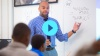## Assessment

The following assessments accompany Unit 3.

### Pre-Unit

Have students complete the Pre-Unit Assessment and Pre-Unit Student Self-Assessment before starting the unit. Use the Pre-Unit Assessment Analysis Guide to identify gaps in foundational understanding and map out a plan for learning acceleration throughout the unit.

### Mid-Unit

Have students complete the Mid-Unit Assessment after lesson 10.

### Post-Unit

Use the resources below to assess student understanding of the unit content and action plan for future units.

Expanded Assessment Package

Use student data to drive your planning with an expanded suite of unit assessments to help gauge students’ facility with foundational skills and concepts, as well as their progress with unit content.

## Unit Prep

### Intellectual Prep

Unit Launch

Prepare to teach this unit by immersing yourself in the standards, big ideas, and connections to prior and future content. Unit Launches include a series of short videos, targeted readings, and opportunities for action planning.#### Intellectual Prep for All Units

• Read and annotate “Unit Summary” and “Essential Understandings” portion of the unit plan.
• Do all the Target Tasks and annotate them with the “Unit Summary” and “Essential Understandings” in mind.
• Take the Post-Unit Assessment.

#### Unit-Specific Intellectual Prep

 Models for up to 4-digit by 1-digit division Written numerical work Base ten block array (can be concrete or pictorial):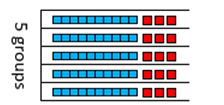Horizontally written partial quotients: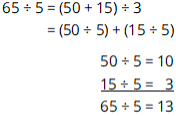Area model with inefficient partial quotients: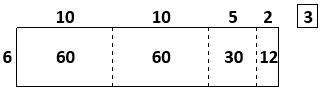Partial quotients algorithm with inefficient partial quotients: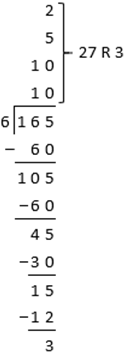Area model with most efficient partial quotients: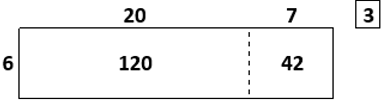Partial quotients algorithm with efficient partial quotients: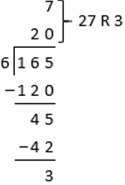Standard algorithm/long division: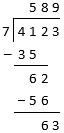### Essential Understandings

• Many division problems involve remainders, which oftentimes require interpretation depending on the context of the problem.
• General methods for division, including the standard algorithm, rely on two main understandings: (1) “quotients of multiples of 10, 100, or 1000 and one-digit numbers” and (2) “the idea of decomposing the dividend into like base-ten units and finding the quotient unit by unit, starting with the largest unit and continuing on to smaller units” (NBT Progression, p. 16).
• While the most efficient way to solve a division problem involves finding the greatest multiple less than the divisor for each place value starting with the largest one, there are many ways in which partial quotients can be computed. For example, to solve $$948\div4$$, one might compute with the greatest multiple less than the divisor for each place value starting with the largest one to get the partial quotients $$(800 \div 4) + (120 \div 4) + (28 \div 4)$$ or one might use less efficient partial quotients, such as $$(400 \div 4) + (400 \div 4) + (80 \div 4) + (40 \div 4) + (20 \div 4) + (8 \div 4)$$, among many other possibilities.
• Unlike the algorithms for other operations, the division algorithm starts with the largest place value first. That is because it is most efficient to do so. For example, when dividing $$34 \div 2$$, if one divided $$4 \div 2$$  then $$30 \div 2$$, the $$30 \div 2$$ necessitates regrouping 1 ten to be 10 ones, resulting in division of ones twice.
• Using inverse operations can help one determine whether an answer to a division problem is reasonable based on whether the quotient times the divisor (plus the remainder, if applicable) results in the dividend.
• Making sense of problems and persevering in solving them is an important practice when solving word problems. Key words do not always indicate the correct operation.

### Materials

• Optional: Square inch tiles (15 per student or small group) — These could be some other object, such as counters. See Lesson 15 Anchor Task 1 Notes for more information.
• Optional: Base ten blocks (Maximum of 9 thousands, 9 hundreds, 9 tens, 20 ones per student or small group) — Students might not need these depending on their reliance on concrete materials. See Lesson 3 Anchor Task 1 Notes for more information.

### Vocabulary

partial quotient

remainder

To see all the vocabulary for Unit 3, view our 4th Grade Vocabulary Glossary.

## Unit Practice

Word Problems and Fluency Activities

Access daily word problem practice and our content-aligned fluency activities created to help students strengthen their application and fluency skills.## Lesson Map

Topic A: Understanding and Interpreting Remainders

Topic B: Division of up to Four-Digit Whole Numbers by One-Digit Whole Numbers

Topic C: Multi-Step Word Problems and Patterns

## Common Core Standards

Key

Major Cluster

Supporting Cluster

### Core Standards

#### Measurement and Data

• 4.MD.A.3 — Apply the area and perimeter formulas for rectangles in real world and mathematical problems. For example, find the width of a rectangular room given the area of the flooring and the length, by viewing the area formula as a multiplication equation with an unknown factor.

#### Number and Operations in Base Ten

• 4.NBT.B.6 — Find whole-number quotients and remainders with up to four-digit dividends and one-digit divisors, using strategies based on place value, the properties of operations, and/or the relationship between multiplication and division. Illustrate and explain the calculation by using equations, rectangular arrays, and/or area models.

#### Operations and Algebraic Thinking

• 4.OA.A.3 — Solve multistep word problems posed with whole numbers and having whole-number answers using the four operations, including problems in which remainders must be interpreted. Represent these problems using equations with a letter standing for the unknown quantity. Assess the reasonableness of answers using mental computation and estimation strategies including rounding.
• 4.OA.C.5 — Generate a number or shape pattern that follows a given rule. Identify apparent features of the pattern that were not explicit in the rule itself. For example, given the rule "Add 3" and the starting number 1, generate terms in the resulting sequence and observe that the terms appear to alternate between odd and even numbers. Explain informally why the numbers will continue to alternate in this way.

• 3.MD.C.5
• 3.MD.C.6
• 3.MD.C.7
• 3.MD.C.7.B
• 3.MD.D.8

• 4.NBT.A.1
• 4.NBT.B.4
• 4.NBT.B.5

• 3.OA.A.1
• 3.OA.A.2
• 3.OA.A.3
• 3.OA.A.4
• 3.OA.B.5
• 3.OA.B.6
• 3.OA.C.7
• 3.OA.D.8
• 3.OA.D.9

• 5.NBT.B.6

• 5.OA.B.3

### Standards for Mathematical Practice

• CCSS.MATH.PRACTICE.MP1 — Make sense of problems and persevere in solving them.

• CCSS.MATH.PRACTICE.MP2 — Reason abstractly and quantitatively.

• CCSS.MATH.PRACTICE.MP3 — Construct viable arguments and critique the reasoning of others.

• CCSS.MATH.PRACTICE.MP4 — Model with mathematics.

• CCSS.MATH.PRACTICE.MP5 — Use appropriate tools strategically.

• CCSS.MATH.PRACTICE.MP6 — Attend to precision.

• CCSS.MATH.PRACTICE.MP7 — Look for and make use of structure.

• CCSS.MATH.PRACTICE.MP8 — Look for and express regularity in repeated reasoning.

Unit 2

Multi-Digit Multiplication

Unit 4

Fraction Equivalence and Ordering

## Request a Demo

See all of the features of Fishtank in action and begin the conversation about adoption.

Yes

No

### Any other information you would like to provide about your school?